# The Imperfection Ratio

A demand vector for a graph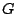with node set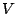is a non-negative vector of integers indexed by nodes of. Given a graphand a demand vector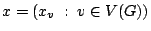a coloring of the pair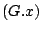is an assignment of a set of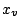colors to each node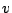ofsuch that two adjacet nodes receive disjoint sets of colors. Coloring the pair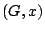corresponds exactly to usual proper coloring of the replicated graph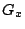. Letbe a graph.Define the imperfection ratio ofby setting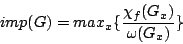where the maximum is over all non-zero integral demand vectors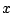and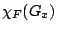and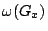are the fractional chromatic number and the clique number of the replicated graphrespectively. (The ratios on the right-hand side above do indeed attain a maximum value). Observe that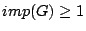.

The next result establishes the connection of the imperfection ratio with perfection.

Proposition For any graph,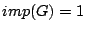iffis perfect.

One of the motivations for studying graph imperfection is its connection to frequency assignment. With this motivation in mind one is particularly interested in bounding the imperfection ratio for graphs of relevant graph classes. One relevant graph class are for example unit disk graph, that is graphs the node set of which can be represented by unit size disks such that two nodes are adjacent if and only if the corresponding disks intersect. It is known that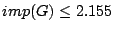for any unit disk graph, and that there exists a unit disk graphwith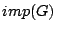arbitrarily close to 3/2.

Conjecture: For any unit disk graph,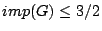.

A subclass of unit disk graphs are the induced subgraphs of the triangular lattice. These graphs are of importance for channel assignment, since a pattern of omni-directional transmitters in two dimensions laid out like nodes of the triangular lattice in the plane give good coverage.

Let us now consider an induced subgraphof the triangular lattice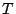. Such a graph has a natural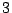-coloring. It is possible to have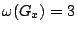and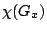=4 for such a graph. There is a polynomial-time coloring algorithm (by McDiarmid and Reed) which shows that for such a graphwe always have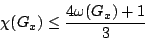Thus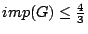for any finite induced subgraphof the triangular lattice. The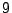-cycle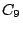is an induced subgraph of the triangular lattice. For any integer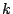the graph obtained fromby replicating each of its nodestimes has clique number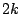and chromatic number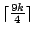Is the ratio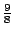of chromatic number to clique number asymptotically the worst (greatest) possible with large demands? This questions may be rephrased in terms ofas follows:

Conjecture For any induced subgraphof the triangular lattice, we have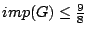This would imply the following weaker and perhaps more tractable conjecture:

Conjecture Ifis a trianlgle-free induced subgraph of the triangular lattice then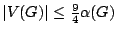(where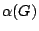is the size of the maximum stable set in), and indeed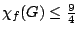To get a feeling for the behavior of the imperfection ratio, we mention the following elementary decomposition result: ifis composed of two parts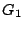and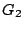that are either disjoint or overlap in a clique, then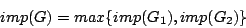The following is another property of imperfection which is desirable for any graph invariant related to perfection:

Proposition For any graph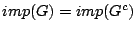where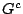denotes the completemt of.

Another graph class of interest are planar graphs. It follows from the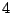-colour theorem that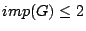for any planar graph. It is known that one can improve a little on this but we conjecture that the true value is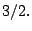Conjecture: For any planar graph,.

Another area of interest are complexity issues concerning imperfection. It is known that it is NP hard to determine the imperfection ratio. One open question is whether for a fixedone can determine in polynomial time whether a graphsatisfies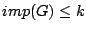. For the special case of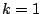, this is the recognition problem for perfect graphs. Another open question is how hard is it to approximate the imperfection ratio of a graph.

Initial contribution by Bruce Reed, extended by Stefanie Gerke

Back to the main index for Perfect Graphs.